• 一般在高维的情况下，如果我们想对后面的维度进行操作，使用负数的索引会更加的方便，在变换张量的维度、计算张量的统计信息，以及张量元素之间进行运算时，都有可能用到轴的概念。 增加维度 在tensorflow中，可以...
张量的存储和视图我们有4个班级的同学，每个班又分为5个小组，每个小组内有10个人，按照从1~10进行编号，这就是逻辑组织。
如果我们把所有的同学们都排成一队，虽然在物理上看起来是一维的，但是在逻辑上仍然是按照班级小组来组织管理的，我们可以通过班级号.组号.组内序号的方式来唯一指定任何一位同学，例如这是2班3组的第6位同学。现在，如果我们将这4个班合并成两个班，每个班10组，那么我们只需要修改学生的班级号和组号就可以了，也就是说逻辑上的形状发生了改变，但是同学们的物理位置保持不变。
同样，当多维张量进行维度变换时，很多时候也只是改变了逻辑上的索引方式，它在内存中的存储情况并没有发生任何改变，我们把张亮的逻辑组织方式称为视图，而把他在内存中的物理组织方式称为存储。
改变张量的形状
tf.reshape(tensor, shape)

tensor是要改变的张量对象， shape是要改变的形状，可以是元组或列表
import tensorflow as tf

a = tf.range(24)
b = tf.reshape(a, [2, 3, 4])
"""
<tf.Tensor: id=5, shape=(2, 3, 4), dtype=int32, numpy=
array([[[ 0,  1,  2,  3],
[ 4,  5,  6,  7],
[ 8,  9, 10, 11]],
[[12, 13, 14, 15],
[16, 17, 18, 19],
[20, 21, 22, 23]]])>
"""

为了更加简洁，可以首先生成一个三维的numpy数组，再用constant转成tensor
tf.constant(np.arange(24).reshape(2, 3, 4))
"""
<tf.Tensor: id=6, shape=(2, 3, 4), dtype=int32, numpy=
array([[[ 0,  1,  2,  3],
[ 4,  5,  6,  7],
[ 8,  9, 10, 11]],
[[12, 13, 14, 15],
[16, 17, 18, 19],
[20, 21, 22, 23]]])>
"""
tf.reshape(b, [4, -1])
"""
<tf.Tensor: id=8, shape=(4, 6), dtype=int32, numpy=
array([[ 0,  1,  2,  3,  4,  5],
[ 6,  7,  8,  9, 10, 11],
[12, 13, 14, 15, 16, 17],
[18, 19, 20, 21, 22, 23]])>
"""

shape参数=-1：自动给推导出长度。
多维张量的轴：张量的维度轴也可以是负数，表示从后向前索引。一般在高维的情况下，如果我们想对后面的维度进行操作，使用负数的索引会更加的方便，在变换张量的维度、计算张量的统计信息，以及张量元素之间进行运算时，都有可能用到轴的概念。
增加维度
在tensorflow中，可以使用tf.expand_dims(input, axis)函数，给张量增加一个维度，其中参数input是输入的张量参数，axis指明要在哪个轴上增加，维度增加的这个维度上，长度为1。
例如创建一个一维的张量，它的形状是2，那么使用tf.expand_dims(input, axis)函数，如果参数x=1就是指增加的维度，在序号为1的轴上因此增加维度后，张量的形状是(2, 1)。下面是三维数组的例子删除维度
tf.squeezr(input, axis)

只能删除长度为1的维度。 input是输入张量，axis是要删除的轴的索引号。如果axis参数省略，会自动删除所有长度为1的维度。增加维度和删除维度，只是改变了张量的视图，不会改变张量的存储。
交换维度
tf.transponse(a, perm)

对于二维张量，就是矩阵的转置。参数perm是各个轴的顺序，可以通过perm调整轴的顺序，从而改变张量的形状。将(0, 1)变成了(1, 0)，也就是进行了转置交换维度，不仅改变了张量的视图，同时也改变了张量的存储顺序。
拼接和分割
拼接张量
tf.concat(tensors, axis)

参数tensors是一个列表，包含所有需要拼接的张量， axis指定在哪个轴上进行拼接，拼接不会产生新的维度。同样，这里axis也可以是负数，-1表示最后一个维度的索引。
分割张量
将一个张量拆分成多个张量，分割后维度不变。
tf.split(tensors, num_or_size_splits, axis=0)

tensors：待分割的张量axis：指明按照哪个轴分割，默认值为0num_orsize_splits：分割的方案
当这个值是单个的值时表示切割的份数 2：分割成两个张量当这个值是一个列表时 [1:2:1]：就表示分割成三个张量，长度是1，2，1
在axis=0上分割，分割成两个分割成3个在axis=1上分割，分割成两个图像的分割和拼接，改变了张量的视图，张量的存储顺序并没有改变。
堆叠和分解
堆叠张量
合并张量时，创建一个新的维度
tf.stack(tensors, axis)

tensors：是要堆叠的多个张量 axis：指定插入新维度的位置3个形状为(4,)的张量堆叠， axis=0：(3, 4) axis=1：(4, 3)
分解张量
将张量分解为多个张量 分解后得到的每个张量，和原来的张量相比，维数都少了一维。
tf.unstack(tensor, axis)展开全文python 机器学习
• 对一个张量进行维度变换，但是最终的总数量必须保持不变 import torch import numpy as np a = torch.rand(4,2,28,28) # 四维张量 print(a.shape) # 原始的张量 print(a.view(8,28*28).shape) # 维度转换成一维...
view/reshape 功能完全一样。对一个张量进行维度变换，但是最终的总数量必须保持不变
import torch
import numpy as np
a = torch.rand(4,2,28,28)  # 四维张量
print(a.shape) #  原始的张量
print(a.view(8,28*28).shape) # 维度转换成一维，总大小不变
print(a.view(-1,8)) # -1 表示任意维度（pytorch根据，后面的维度自己推导，如总维度是 28*28*8，此时-1代表的维度就是28*28*8 / 8 =28*28）

torch.Size([4, 2, 28, 28])
torch.Size([8, 784])
torch.Size([784, 8])

suqeeze/unsqueeze 维度的压缩与扩展
unsuqeeze
import torch
import numpy as np
a = torch.rand(4,2,28,28)  # 四维张量
print("a 的初始维度是：{}".format(a.shape)) #  原始的张量
print("a 增加一个维度是：{}".format(a.unsqueeze(0).shape)) # 最前面增加一个维度
print("a 增加一个维度是：{}".format(a.unsqueeze(1).shape)) # 在原来第二个维度前增加一个维度
print("a 增加一个维度是：{}".format(a.unsqueeze(-1).shape)) # 最后面增加一个维度


a 的初始维度是：torch.Size([4, 2, 28, 28])
a 增加一个维度是：torch.Size([1, 4, 2, 28, 28])
a 增加一个维度是：torch.Size([4, 1, 2, 28, 28])
a 增加一个维度是：torch.Size([4, 2, 28, 28, 1])


suqeeze
import torch
import numpy as np
a = torch.rand(4,2,28,28,1,1)  # 四维张量
print("a 的初始维度是：{}".format(a.shape)) #  原始的张量
print("a 删除维度为1的维度是：{}".format(a.squeeze().shape)) # 删除所有维度为1的维度
print("a 删除指定位置为1的维度是：{}".format(a.squeeze(4).shape)) #

a 的初始维度是：torch.Size([4, 2, 28, 28, 1, 1])
a 删除维度为1的维度是：torch.Size([4, 2, 28, 28])
a 删除指定位置为1的维度是：torch.Size([4, 2, 28, 28, 1])

转置
转置 .t() (二维)
import torch
import numpy as np
a = torch.rand(4,2)  # 四维张量
print("a 转置后是：{}".format(a.t().shape))

a 转置后是：torch.Size([2, 4])

transpose 两个维度相互交换
import torch
import numpy as np
a = torch.rand(4,3,32,32)  # 四维张量
#transpose 后需要接 contiguous 保证数据在内存的连续性
a1 = a.transpose(1,3).contiguous().view(4,3*32*32).view(4,3,32,32)
a2 = a.transpose(1,3).contiguous().view(4,3*32*32).view(4,32,32,3).transpose(1,3)
# torch.all 判断所有元素   torch.eq 判断两个张量是否相等
print("a1 shape : {}  a2 shape : {}".format(a1.shape,a2.shape))
# 判断变换后 a 与 a1 是否相同
print("a1 与 a 是否相同：{}".format(torch.all(torch.eq(a,a1))))
# 判断变换后 a 与 a2 是否相同
print("a2 与 a 是否相同：{}".format(torch.all(torch.eq(a,a2))))

a1 shape : torch.Size([4, 3, 32, 32])  a2 shape : torch.Size([4, 3, 32, 32])
a1 与 a 是否相同：False
a2 与 a 是否相同：True

permute 任意维度的交换
import torch
import numpy as np
a = torch.rand(4,3,32,32)  # 四维张量
#permute 后需要接 contiguous 保证数据在内存的连续性
a1 = a.permute(0,3,2,1).contiguous() # 按各维度的索引进行排列
print("a1 shape : {} ".format(a1.shape))

a1 shape : torch.Size([4, 32, 32, 3])

展开全文机器学习 python 深度学习
• np.transpose()将numpy矩阵的纬度按[2,0,1]矩阵交换，np.expand_dims()维度拓展一个维度，位置为axis = 1,维度。一般直接用.copy()进行矩阵复制，不要直接相等。 rgb = np.expand_dims(np.transpose(rgb,[2,0,1]),...
np.transpose()将numpy矩阵的纬度按[2,0,1]矩阵交换，np.expand_dims()维度拓展一个维度，位置为axis = 1,维度。一般直接用.copy()进行矩阵复制，不要直接相等。
    rgb = np.expand_dims(np.transpose(rgb,[2,0,1]),axis=1).copy()

“RuntimeError: Input type (torch.cuda.DoubleTensor) and weight type (torch.cuda.FloatTensor) should be the same”错误解决办法
    input = torch.from_numpy(rgb)
input = input.cuda()
target = target.cuda()

因为pytorch中的weight的类型存储为float32存储，而input为double(64)位，所以input和weight都为cuda()型的。并且类型要一样，但是numpy自动存储的类型为double(Float32)，所以要将input的类型调整为double()型。
    input = torch.from_numpy(rgb)
input = input.type(torch.FloatTensor)
input = input.cuda()
target = target.cuda()

将tensor转化为numpy，转化为数值。当转化的时候要先转化为Tensor.cpu()，在转化为Tensor.numpy()
testLoss = testLoss.cpu().numpy()

展开全文numpy 人工智能 cuda 深度学习
• 1.维度变换 import tensorflow as tf import numpy as np print(tf.__version__) # 维度变换 a = tf.random.normal([4, 28, 28, 3]) print(a.shape) # 维度变换 [4, 28, 28, 3] --> [4, 28 * 28, 3] print(tf....
1.维度变换
import tensorflow as tf
import numpy as np
print(tf.__version__)

# 维度变换
a = tf.random.normal([4, 28, 28, 3])
print(a.shape)

# 维度变换 [4, 28, 28, 3] --> [4, 28 * 28, 3]
print(tf.reshape(a, [4, 784, 3]).shape)
# 维度变换 [4, 28, 28, 3] --> [4, 784 * 3]
print(tf.reshape(a, [4, -1]).shape)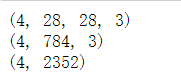2.矩阵的转置
# 矩阵的转置
a = tf.random.normal((4, 3, 2, 1))
print(a.shape)
# 维度真实颠倒 [4, 3, 2, 1] ==> [1, 2, 3, 4]
print(tf.transpose(a).shape)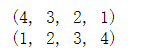a = tf.random.normal((4, 28, 28, 3))
print(a.shape)
# 根据需要 转置指定维度
# 维度真实颠倒 [b, h, w, c] --> [b, c, h, w]
print(tf.transpose(a, [0, 3, 1, 2]).shape)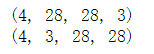3.增加 展开维度
# 增加 展开维度
a = tf.random.normal([4, 35, 8])
print(a.shape)
# 前面增加一个维度
print(tf.expand_dims(a, axis=0).shape)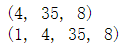# 后面增加一个维度
print(a.shape)
print(tf.expand_dims(a, axis=3).shape)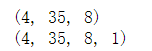4.压缩维度
# 压缩维度
a = tf.zeros([1, 2, 1, 1 ,3])
print(a.shape)
# 压缩所有1维维度
print(tf.squeeze(a).shape)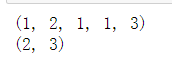# 压缩指定维度
print(a.shape)
print(tf.squeeze(a, axis=2).shape)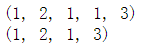展开全文• 1：增加维度 x[np.newaxis, :, :] 将x从两维增加成三维，np.newaxis放在第一个表示在第一维增加 2：变换轴的维度 x.transpose(2, 0, 1) 表示将三个维度的顺序调换
• ## pytorch维度变换

千次阅读 2019-05-12 19:18:00
#维度变换1:view容易造成数据存储方式丢失. a = torch.rand(4,1,28,28) print(a.shape,a.view(4,28,28))#4,28,28 4张图片,把每张图片都合并在一起,即784,常用于全连接层; print(a.view(4,28*28).shape)#...
• 维度图像维度变换 当图像对应的矩阵的维度为[64, ...为了将矩用于Tensorflow的输入格式，即4000×64×64×1，使用np.transpose((3, 0,1, 2))来进行变换： sc_test = sc_test.transpose((3, 0, 1, 2)) slope_tes...
•tensorflow
• a=arange(12) a.reshape(4,3)转换为4*3的二维数组  b=np.random.randint(1,6,(3,4)) b.reval()变为一维向量 a=np.arange(4) ...b=a[:,np.newaxis]在列上添加一个维度，变成4*1 out:array([, ...
• np.transpose(input, axes=None) 2. np.concatenate((a1, a2, …), axis=0) 3. np.newaxis 实践出真知 1. 扩展出一个新的维度用来表示channel 2.叠加channel 3.维度转置 4.验证是否正确 5.整体演示...
• np a = np . arange ( 8 ) print ( '原始数组：' ) print ( a ) print ( '\n' ) b = a . reshape ( 4 , 2 ) print ( '修改后的数组：' ) print ( b ) 原始数组： [ 0 1 2 3 ...numpy
• 文章目录维度变换简介ViewReshapeReshape is flexib 重塑是灵活的Reshape could lead to potential bugs!tf.transpo 矩阵转置[b,h,w,c]→[b, 3, h, w]Squeeze VS Expand_dimsExpand dimSqueeze dim 维度变换 简介 ...tensorflow
• 1.np，tensor互相转换 要对tensor进行操作，需要先启动一个Session,否则，我们无法对一个tensor比如一个tensor常量重新赋值或是做一些判断操作，所以如果将它转化为numpy数组就好处理了。这里以一副图像为例： 2...tensorflow axis
• np.fft.fft进行快速傅里叶变换，需要特别注意的是，默认是对最后个维度的数据进行fft变换，若你输入的是一维数组，请忽略后面，如果你是二维列矢量，如果不将数据变为行矢量或将axis参数设为0，将得到与输入相同的...python
• 注意：使用cv2.imread()读取彩色图片时，OpenCV获得的张量的数据顺序为h*w*c，其中张量的最后一个维度才是通道，所以在送入到torch中之前，需要对张量的维度顺序进行变换； 一个可行的方式为 img = img.transpose...OpenCV
• 维度变换 tf.reshape ：改变张量形状 a = tf.random.uniform(shape=[1,3,3,2],minval=0,maxval=255,dtype=tf.int32) # 将原tensor reshape为3行6列的tensor tf.reshape(a,[3,6]) tf.squeeze：减少维度，去掉一维...tensorflow
• 不足之处：会丢失维度信息（Lost dim information） import torch import numpy as np #创建Tensor def test(): a = torch.rand(4, 1, 28, 28) print(a.shape) #[4, 1*28*28] print(a.view(4, 1*28*28)) ...python...